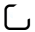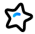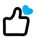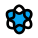# 终于实现了一门属于自己的编程语言

crossoverJie2022年9月9日
• 微信公众号## # 特性

hello_world.gs:

``````println("hello world");
``````
``````❯ gscript hello_world.gs
hello world
``````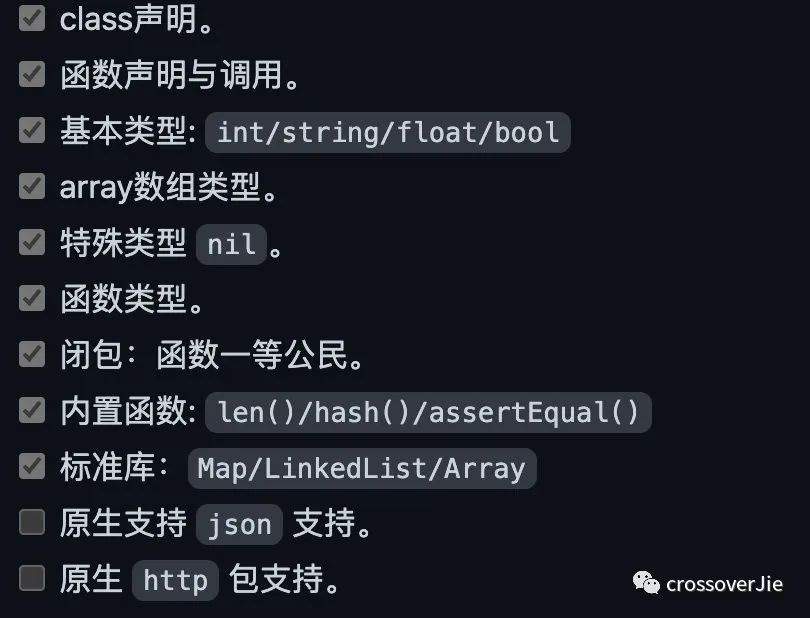## # 例子

``````void fib(){
int a = 0;
int b = 1;
int fibonacci(){
int c = a;
a = b;
b = a+c;
return c;
}
return fibonacci;
}
func int() f = fib();
for (int i = 0; i < 5; i++){
println(f());
}
``````

``````0
1
1
2
3
``````

``````// fib returns a function that returns
// successive Fibonacci numbers.
func fib() func() int {
a, b := 0, 1
return func() int {
a, b = b, a+b
return a
}
}
func main() {
f := fib()
// Function calls are evaluated left-to-right.
fmt.Println(f(), f(), f(), f(), f())
}
``````

## # 语法

`GScript` 的语法与常见的 `Java/Go` 类似，所以上手非常简单。

### # 基本类型

``````int a=10;
string b,c;
float e = 10.1;
bool f = false;
``````

### # 数组

``````// 声明并初始化
int[] a={1,2,3};
println(a);

// 声明一个空数组并指定大小
int[] table = {};

println();
// 向数组 append 数据
a = append(a,4);
println(a);
for(int i=0;i<len(a);i++){
println(a[i]);
}

// 通过下标获取数组数据
int b=a;
println(b);
``````

``````int[] a={};
println("数组大小:"+len(a));
a = append(a,1);
println("数组大小:"+len(a));
println(a);
a=100;
println(a);
``````

``````数组大小:2

[<nil> <nil> 1]
[100 <nil> 1]
``````

### # Class

``````class ListNode{
int value;
ListNode next;
ListNode(int v, ListNode n){
value =v;
next = n;
}
}

// 调用构造函数时不需要使用 new 关键字。
ListNode l1 = ListNode(1, nil);

// 使用 . 调用对象属性或函数。
println(l1.value);
``````

``````class Person{
int age=10;
string name="abc";
int getAge(){
return 100+age;
}
}

// 无参构造函数
Person xx= Person();
println(xx.age);
assertEqual(xx.age, 10);
println(xx.getAge());
assertEqual(xx.getAge(), 110);
``````

``````// 大小为 16 的 Person 数组
Person[] personList = {};
``````

### # 函数

• 普通的全局函数。
• 类的函数。

``````// 判断链表是否有环
return false;
}
return false;
}

bool ret = false;
for (fast.next != nil){
if (fast.next == nil){
return false;
}
if (fast.next.next == nil){
return false;
}
if (slow.next == nil){
return false;
}
if (fast == slow){
ret = true;
return true;
}

fast = fast.next.next;
slow = slow.next;
}
return ret;
}

ListNode l1 = ListNode(1, nil);
bool b1 =hasCycle(l1);
println(b1);
assertEqual(b1, false);

ListNode l4 = ListNode(4, nil);
ListNode l3 = ListNode(3, l4);
ListNode l2 = ListNode(2, l3);
bool b2 = hasCycle(l2);
println(b2);
assertEqual(b2, false);

l4.next = l2;
bool b3 = hasCycle(l2);
println(b3);
assertEqual(b3, true);
``````

``````add(int a){}
``````

### # 闭包

``````// 外部变量，全局共享。
int varExternal =10;
func int(int) f1(){
// 闭包变量对每个闭包单独可见
int varInner = 20;
int innerFun(int a){
println(a);
int c=100;
varExternal++;
varInner++;
return varInner;
}
// 返回函数
return innerFun;
}

// f2 作为一个函数类型，接收的是一个返回值和参数都是 int 的函数。
func int(int) f2 = f1();
for(int i=0;i<2;i++){
println("varInner=" + f2(i) + ", varExternal=" + varExternal);
}
println("=======");
func int(int) f3 = f1();
for(int i=0;i<2;i++){
println("varInner=" + f3(i) + ", varExternal=" + varExternal);
}
``````

``````0
varInner=21, varExternal=11
1
varInner=22, varExternal=12
=======
0
varInner=21, varExternal=13
1
varInner=22, varExternal=14
``````
``````func int(int) f2 = f1();
``````

## # 标准库

### # MapString

``````int count =100;
MapString m1 = MapString();
for (int i=0;i<count;i++){
string key = i+"";
string value = key;
m1.put(key,value);
}
println(m1.getSize());
assertEqual(m1.getSize(),count);

for (int i=0;i<count;i++){
string key = i+"";
string value = m1.get(key);
println("key="+key+ ":"+ value);
assertEqual(key,value);
}
``````

### # 内置函数

``````int[] a={1,2,3};
// len 返回数组大小
println(len(a));

// 向数组追加数据
a = append(a,4);
println(a);
// output: [1,2,3,4]

// 断言函数，不相等时会抛出运行时异常，并中断程序。
assertEqual(len(a),4);

// 返回 hashcode
int hashcode = hash(key);
``````

## # 总结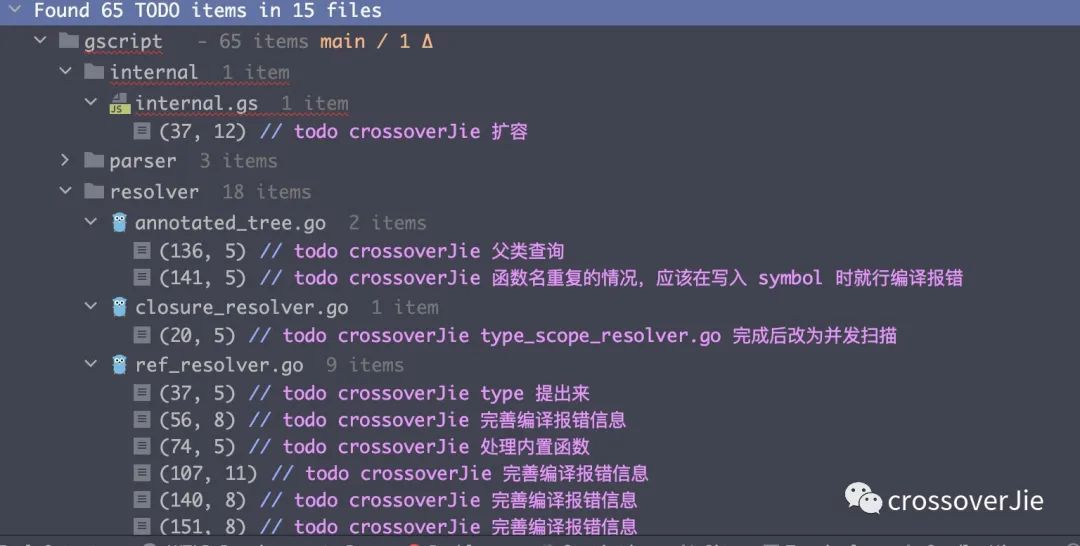[

2022-08-17[

2022-08-08[

2022-08-01[

2022-06-28